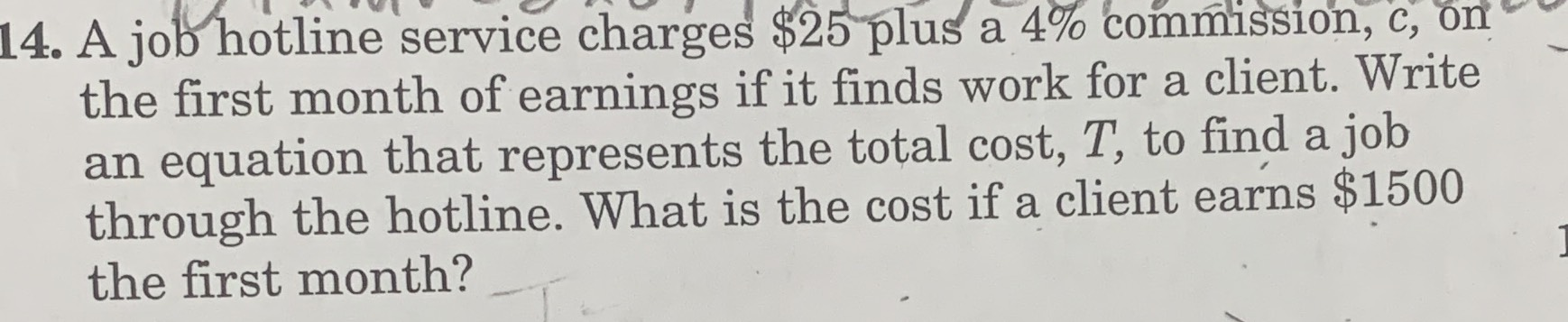### ¿Todavía tienes preguntas de matemáticas?

Pregunte a nuestros tutores expertos
Algebra
Pregunta14. A joo hotline service charges $$\ 25$$ plus a $$4 \%$$ commission, c, on the first month of earnings if it finds work for a client. Write an equation that represents the total cost, $$T$$ , to find a job through the hotline. What is the cost if a client earns $$\ 1500$$ the first month?

T = $25 +0.04c T =$25 + 0.04(1500) =\$85